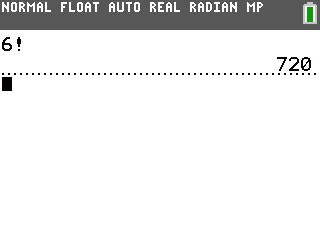# Knowledge Base

## Solution 34652: Calculating Factorials (!) Using the TI-83 Plus and TI-84 Plus Family of Graphing Calculators.

### How do I calculate a factorial using the TI-83 Plus and TI-84 Plus Family of graphing calculators?

The instructions below display how to calculate a factorial.

Example: Find 6!.

• On the home screen, press [CLEAR] to clear any previous entries.
• Press  [MATH] and the left arrow key to select the PROB menu.
• Press  for !.
• Press [ENTER] to display the answer of 720.NOTES:

• The factorial is computed recursively using the relationship (n+1)! = n*n! until n is reduced to either 0 or -1/2. At that point, the definition 0!=1 or the definition (-1/2)!= Öp is used to complete the calculation.

! (factorial) returns the factorial of either an integer or a multiple of 0.5. For a list, it returns factorials for each integer or multiple of 0.5. The factorial value must be greater than or equal to -0.5 and less than or equal to 69.

Please see the TI-83 Plus and TI-84 Plus Family guidebooks for additional information.